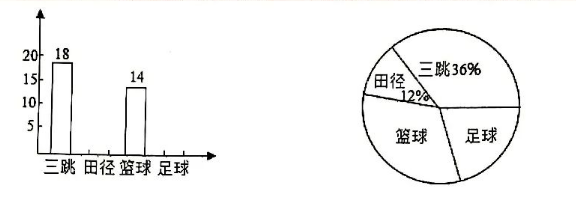（1）请你通过计算补全条形统计图;
(2)请你求出的形统计图中 “最喜欢足球 ${ }^n$ 对应的扇形图心角度数.
（3）请你估计全校 “最喜欢篮球” 的总人数.【答案】 $18 \div 36 \%=50$ (人

（1）略
（2）$360^{\circ} \times \frac{14}{50}=86.4^{\circ}$
（3）$3200 \times \frac{14}{50}=896(\alpha)$